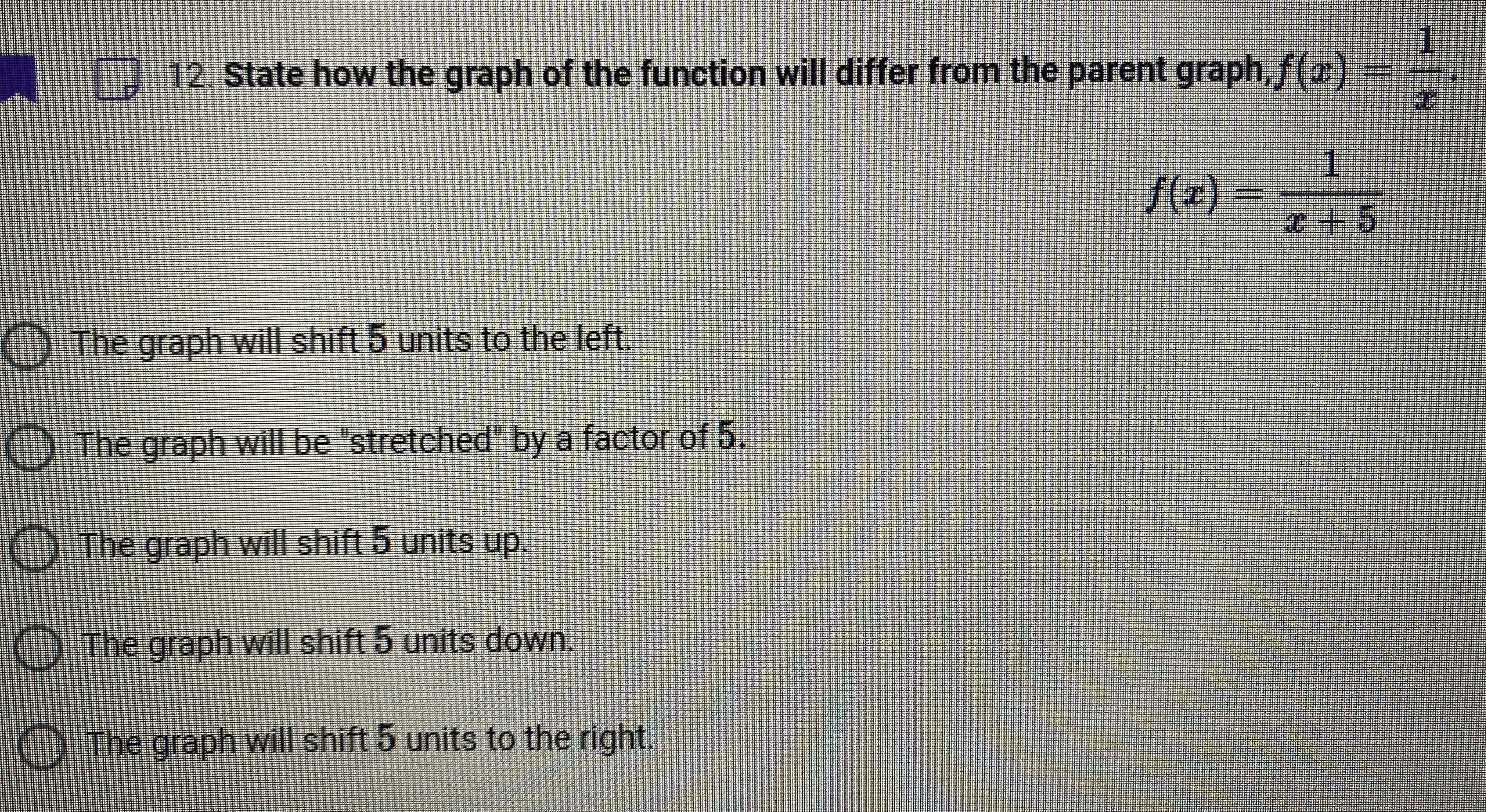### ¿Todavía tienes preguntas de matemáticas?

Pregunte a nuestros tutores expertos
Algebra
Pregunta12. State how the graph of the function will differ from the parent graph, $$f ( x ) = \frac { 1 } { x }$$

$$f ( x ) = \frac { 1 } { x + 5 }$$

The graph will shift $$5$$ units to the left.

The graph will be "stretched" by a factor of $$5 .$$

The graph will shift $$5$$ units up.

The graph will shift $$5$$ units down.

The graph will shift $$5$$ units to the right.